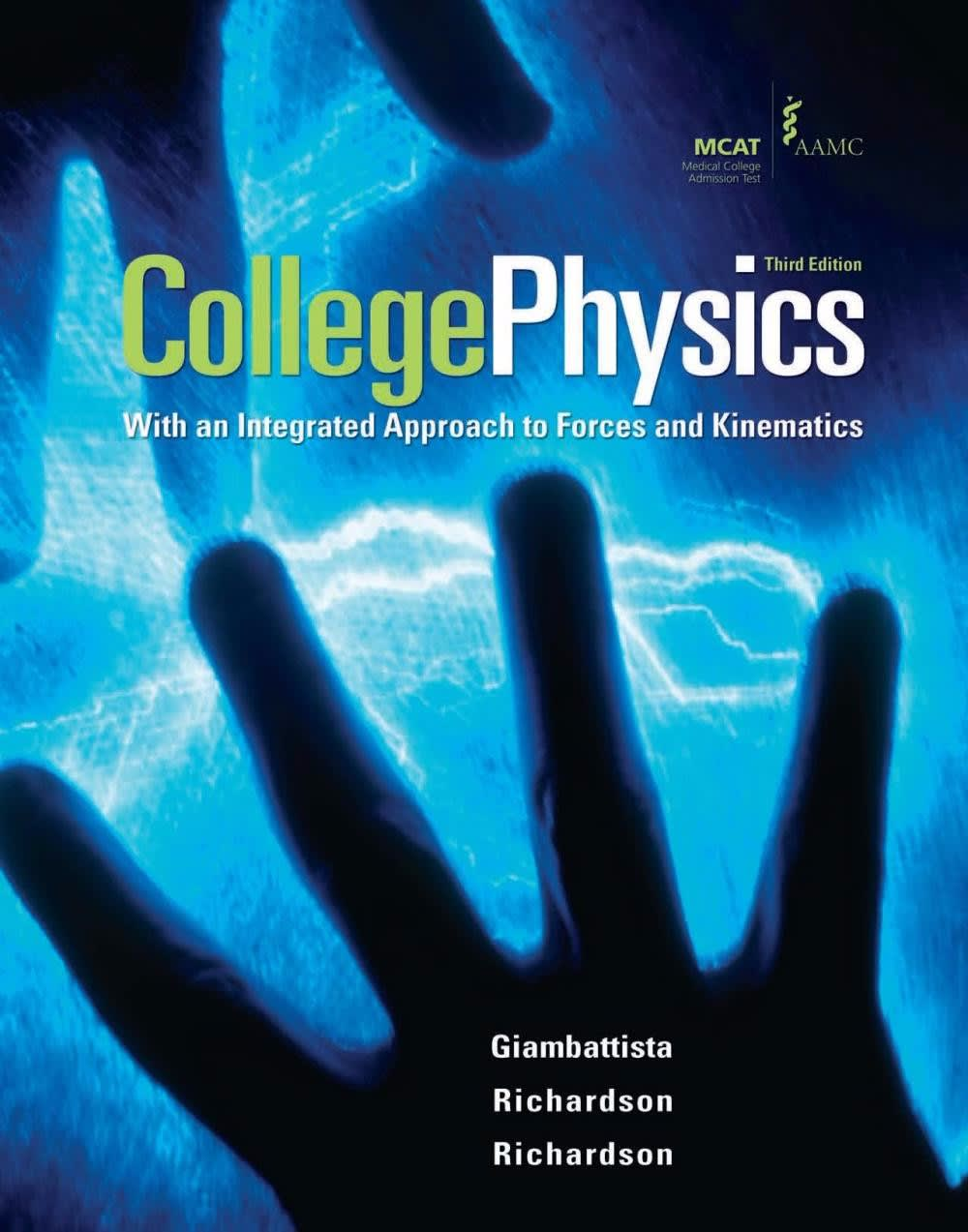Physics College Physics# College Physics Giambattista • 3rd Edition • 978-0077263218

Not the textbook you were looking for? Pick another one here.

## Ch 01: Introduction

Introduction to Units Unit Conversions Dimensional Analysis Counting Significant Figures Operations with Significant Figures

## Ch 03: Acceleration and Newton's Second Law of Motion

Vectors, Scalars, & Displacement Average Velocity Intro to Acceleration

## Ch 04: Motion with Constant Acceleration

Force Problems with Motion Forces with Multiple Objects Vertical Forces & Acceleration Projectile Motion More Projectile Motion Initial Velocity in Projectile Motion Forces in 2D

## Ch 10: Elasticity and Oscillations

Spring Force (Hooke's Law) Intro to Simple Harmonic Motion (Horizontal Springs) Energy in Simple Harmonic Motion Simple Harmonic Motion of Vertical Springs Simple Harmonic Motion of Pendulums Energy in Pendulums

## Ch 11: Waves

What is a Wave? The Mathematical Description of a Wave Waves on a String Wave Interference Standing Waves

## Ch 12: Sound

Sound Waves Standing Sound Waves Sound Intensity Beats The Doppler Effect

## Ch 13: Temperature and the Ideal Gas

Temperature Zeroth Law of Thermodynamics Thermal Expansion Introduction to Ideal Gasses Kinetic Theory of Gasses

## Ch 15: Thermodynamics

First Law of Thermodynamics Thermal Processes Four Stroke Piston Engine Carnot Cycle Refrigerators Entropy and the Second Law

## Ch 20: Electromagnetic Induction

Intro to Induction Magnetic Flux Faraday's Law Lenz's Law Motional EMF Transformers Mutual Inductance Self Inductance Inductors LR Circuits

## Ch 22: Electromagnetic Waves

What is an Electromagnetic Wave? The Electromagnetic Spectrum Energy Carried by Electromagnetic Waves Electromagnetic Waves as Sinusoidal Waves Polarization Filters

## Ch 23: Reflection and Refraction of Light

Ray Nature Of Light Reflection Of Light Refraction Of Light Total Internal Reflection Ray Diagrams For Mirrors Mirror Equation Ray Diagrams For Lenses Thin Lens And Lens Maker Equations

## Ch 25: Interference and Diffraction

Diffraction 36.02 (A, n/a): Diffraction with Huygen's Principle Young's Double Slit Experiment Single Slit Diffraction

## Ch 25: Relativity

Inertial Reference Frames Special Vs. Galilean Relativity Consequences of Relativity Lorentz Transformations